homechevron_rightStudychevron_rightMath

# Egyptian numbers

The calculator displays a number in ancient Egypt hieroglyphs. Multiple numbers are recognised as Egyptian fraction.

This calculator displays a number in the form of ancient Egyptian hieroglyphs. The ancient Egyptian numeral system was used since around 3000 B.C.E. during three to four thousands years. The numeral system description can be found just below the calculator.#### Egyptian numbers

Number or space-separated Egyptian fraction coefficients
Hieroglyphs

Number with Egyptian fraction

Number of hieroglyphs

Alternative number of hieroglyphs

### The ancient Egyptian numbering system 1

The ancient Egyptians developed the numbering system which is based on 10, no doubt because human has ten fingers. They had the symbols to represent units, tens, hundreds, thousands, tens of thousands and millions:

Hieroglyph Value Description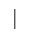1 stroke10 hobble for cattle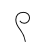100 coil of rope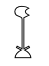1 000 lotus plant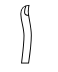10 000 finger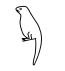100 000 tadpole (polliwog)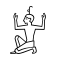1 000 000 god Heh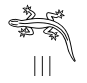Many millions (infinity) lizard with three strokes

The corresponding symbol is simply repeated to represent a number from 2 to 9, several tens, several hundreds and so on.

#### Scribing order

The ancient Egyptians carved or painted most of their hieroglyphs from right to left, but sometimes the scribing order was reversed. Our calculator produces the numerical hieroglyphs sequence in reverse order with higher degree symbols on the left and lower degree ones on the right. You may recognise the reading order by the figures with face - start reading toward the front of its head.

#### Egyptian fractions

The ancient Egyptians scribed a fractional number by a sum of unit fractions. For example number 0.89 (89/100) can be expanded to the sum of unit fractions: 1/2+1/3+1/18+1/900. Read Egyptian fractions for more details.
1/2 was represented by special symbol, the other unit fraction denominators were scribed under the mouth symbol.

Hieroglyph Description1/2 - half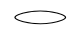the mouth symbol was placed over a unit fraction denominator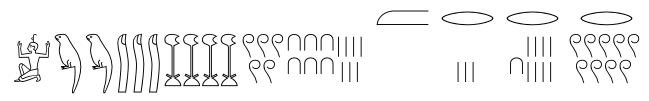The fractional number in ancient Egyptian hieroglyphs. The inscription reads 1 234 567+1/2+1/3+1/18+1/900 (1 234 567.89 in decimal) .

1. Donald Frazer, Hieroglyphs and Arithmetic of the Ancient Egyptian Scribes